## 数据获取

import requests
import pandas as pd
import matplotlib.pyplot as plt
import geopandas as gpd #基于pandas库的用来绘制地图的库
import io
import PIL #处理图像的库

• 注意，geopandas库的安装有点麻烦，不是简单的pip下载安装就能搞定的，请自行百度安装教程。另外如果运行的时候报错提示缺少库，就按照提示安装相应的库。

### 疫情数据集

https://github.com/CSSEGISandData/COVID-19

def getDataFromGithub():
#这个url链接的文件是关于新冠疫情爆发至今，世界上每个国家确诊人数的时间线
url = 'https://raw.githubusercontent.com/CSSEGISandData/COVID-19/master/csse_covid_19_data/csse_covid_19_time_series/time_series_covid19_confirmed_global.csv'
try:
response = requests.get(url)
with open('time_series_covid19_confirmed_global.csv','wb') as f:
f.write(response.content)
except:
print('在线获取失败！')
return -1


def getData():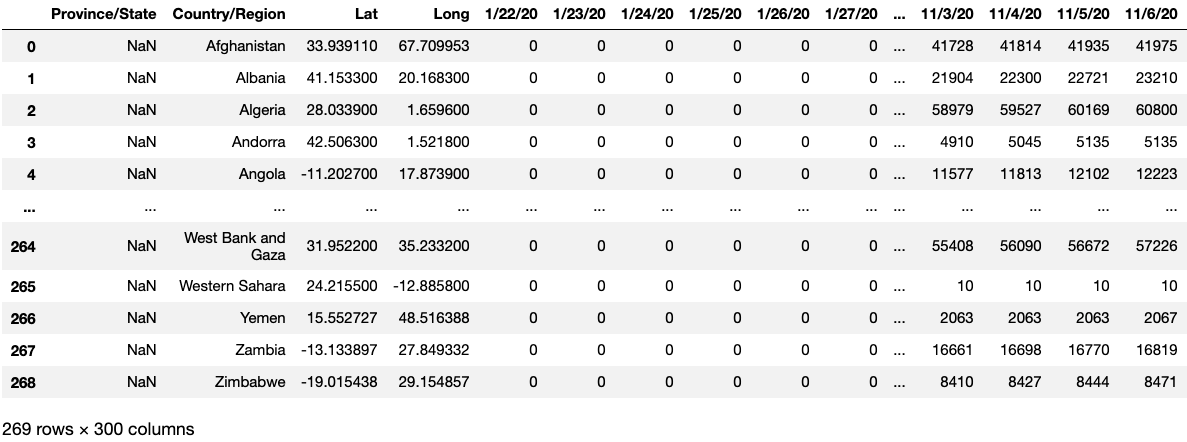### 国家地理数据集

https://github.com/ShallowHui/interesting-small-project

def getGeoData():
return world #返回一个geopandas模块的GeoDataFrame数据类型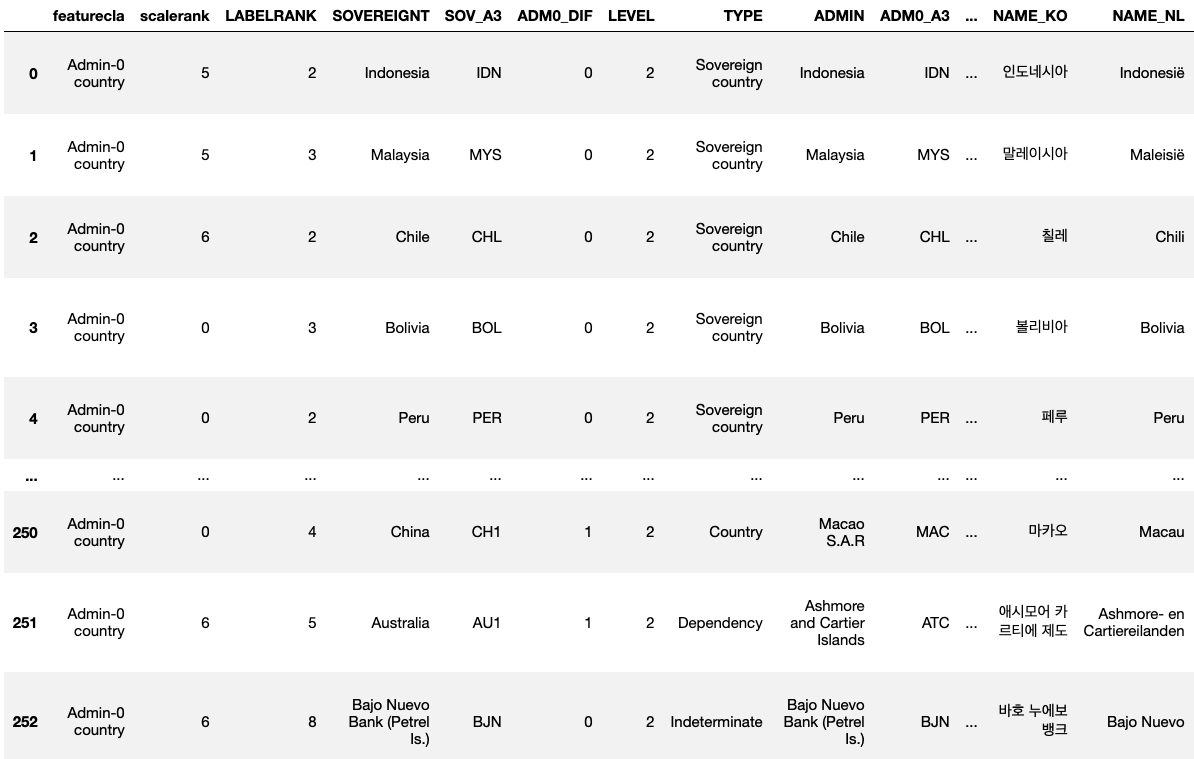## 数据清洗

1. 两个数据集都是把台湾地区单独列出来的：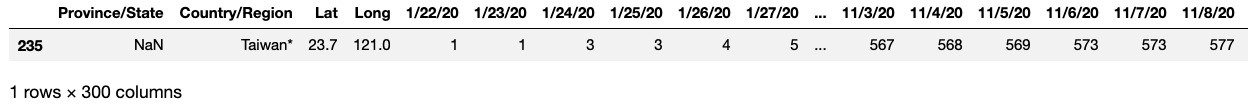1. 两个数据集中的国家名不一定对得上，比如地理数据集中的美国国家名为United States of America，而疫情数据集中却为US

def MergeData(df,countries): #接收df：疫情数据集，countries：国家地理数据集
#将台湾地区设置为中国
df['Country/Region'].replace('Taiwan*','China',inplace=True)
countries['SOVEREIGNT'].replace('Taiwan','China',inplace=True)

#删除疫情数据集中的经纬度数据，因为这在地理数据集中有
df = df.drop(columns=['Lat','Long'])
#因为疫情数据集中有一些国家是按省份、州进行统计的，一国数据分散，所以要把它们合并
df = df.groupby('Country/Region').sum()

#简化地理数据集，只用保留坐标列和主权名即可
countries = countries.drop(columns=[col for col in countries.columns if col not in ['SOVEREIGNT','geometry']])
#然后尽可能地将地理数据集中的国家名改成跟疫情数据集一样
countries['SOVEREIGNT'].replace('United States of America','US',inplace=True)
countries['SOVEREIGNT'].replace('South Korea','Korea, South',inplace=True)
countries['SOVEREIGNT'].replace('Vatican','Holy See',inplace=True)
countries['SOVEREIGNT'].replace('eSwatini','Eswatini',inplace=True)
countries['SOVEREIGNT'].replace('United Republic of Tanzania','Tanzania',inplace=True)

#合并数据集
return countries.join(df,how='right',on='SOVEREIGNT')


df = getData()
countries = getGeoData()
mergeData = MergeData(df,countries)

fig,ax = plt.subplots(figsize=(23,10))
plt.title('Total confirmed cases of COVID-19 in the World') #设置画布标题
plt.text(-35,-30,'11/11/20',fontdict={'size':26 , 'color':'blue'}) #显示当前日期
mergeData.plot(
column = '11/11/20', #绘制哪一列的数据
scheme = 'userdefined', #用户自定义模式
classification_kwds = {'bins':[0,100,1000,10000,100000,1000000]}, #定义分位点，即疫情严重程度
cmap = 'Reds', #颜色
legend = True, #显示图例
legend_kwds = dict(loc='lower left'), #指定图例显示位置
ax = ax #在指定画布的坐标轴上绘制
)
plt.show()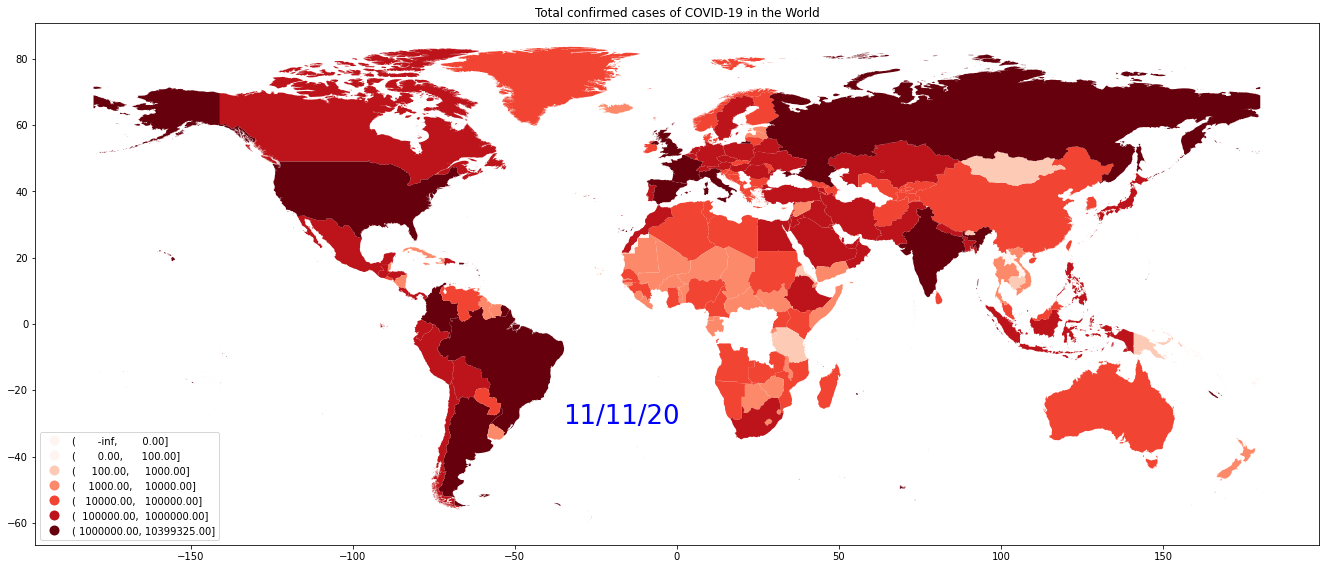## 实现动态可视化

def ImgToGif(data):
GIF = [] #保存gif动图里的每一张图片
dates = data.columns[76:86] #获取数据集中的日期数据
fig,ax = plt.subplots(figsize=(23,10))
sum = 0
length = len(dates)
#循环生成每一天的世界疫情图
for date in dates:
plt.title('Total confirmed cases of COVID-19 in the World, '+date,fontdict={'size':30}) #设置画布标题
data.plot(
column = date, #绘制哪一列的数据
scheme = 'userdefined', #用户自定义模式
classification_kwds = {'bins':[0,100,1000,10000,100000,1000000]}, #定义分位点，即疫情严重程度
cmap = 'Reds', #颜色
legend = True, #显示图例
legend_kwds = dict(loc='lower left'), #指定图例显示位置
ax = ax #在指定画布的坐标轴上绘制
)
img = ax.get_figure() #获取绘制完成后的画布
f = io.BytesIO() #打开IO流
img.savefig(f,format='png') #将图片写入IO流
f.seek(0) #文件指针移回初始位置
GIF.append(PIL.Image.open(f)) #用PIL模块的专门处理图像的子模块读取IO流，并将流式数据转化成PngImageFile数据类型存放到GIF列表中
sum = sum + 1
print('\r生成动图中：{:.2f}%'.format(sum*100./length),end='')

#将列表里的所有图片合并生成GIT图
GIF.save(
'COVID-19_visual.gif',
format='GIF', #保存为GIF动图
append_images=GIF[1:],
save_all=True,
duration=200, #动图时间间隔，单位毫秒
loop=0 #loop=0代表无限循环播放动图
)
f.close()
print('\n\r动图生成完成！')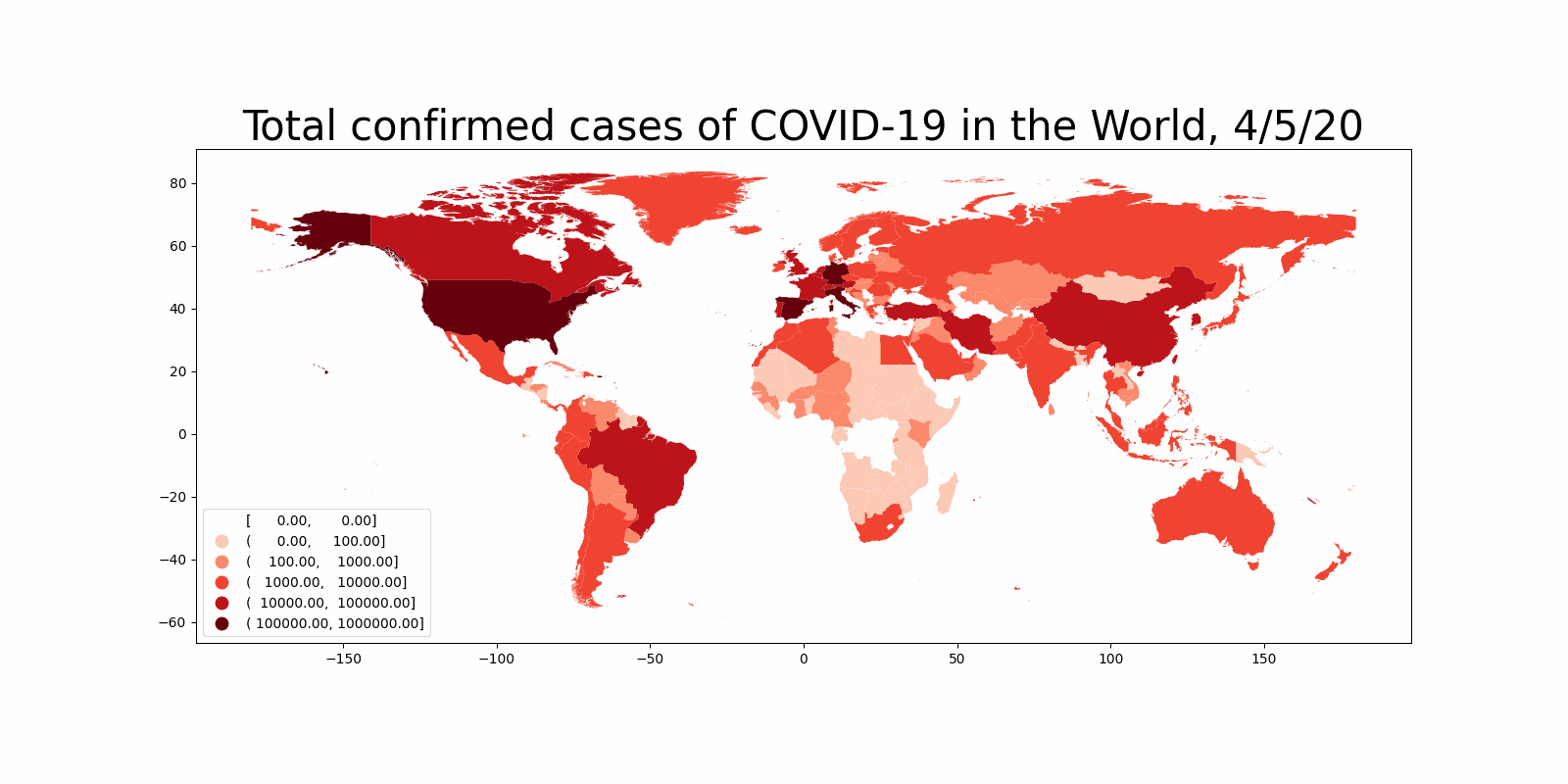## 总结

https://github.com/ShallowHui/interesting-small-project/blob/master/COVID-19_visual.py

• 本代码只适合运行于window平台，在其它平台运行可能会报库不兼容错误。

Q.E.D.

••